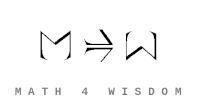文章 发现 ms@ms.lt +370 607 27 665 My work is in the Public Domain for all to share freely. 读物 书 影片 维基百科 Software Upload Understand the relationship between left adjoints and right adjoints, colimits and limits, and internal structure and external relationships. 伴隨函子 Understanding facts about adjunction What is the nature of the relationships between right adjoints (and limits) and left adjoints (and colimits)? How conditions impact adjunction What is a functor that is self-adjoint? What are adjoint functors when {$C=D$}? What are adjoint functors when {$C\cong D$}? What are adjoint functors when F and G are exact inverses? Is it possible to have a three-cycle of adjoint endofunctors {$F\dashv G\dashv H\dashv F$}? Asymmetry of adjoint functors In what sense does adjunction express asymmetry? Intuit the difference between left adjoints and right adjoints. Adjoint functors describe understanding each other: susikalbėjimas. Where does the asymmetry arise on either side? Is it like a parent and a child understanding each other? Adjoint functors and perspectives How do functors express perspectives (or understandings) and their composition? As in "my understanding of her understanding of my understanding" as occurs in adjunctions GF(Y)? What is the relationship between perspectives-understandings and cones, cocones, limits, colimits, left and right adjunctions? Category of perspectives: stepping-in and stepping-out as adjoints? there exists vs. for all? Wholeness preserving What is the relation between Alexander's "wholeness preserving function" and the preservation of limits or colimits? How is the triviality of a functor related to wholeness preserving? Intuitive understanding of adjunction What does Varela mean by complementarity? How does my description of adjunction as expressing communication relate to Shannon theory of information? Adjoint functors expressing my philosophy Where do inverses and approximate inverses come up in my philosophy? Compare adjoint functor with constructive hypothesis. Compare adjoint functor with the pragmatically absolute. Extending the domain Compare adjoint functor with "extension of the domain", extension of structure. How is "extending the domain" related to Kan extensions? How are adjunctions and Kan extensions both like extensions of the domain? Understand how a Kan extension expresses left and right adjunctions, as in Riehl's book 6.5.2, page 210. Try to relate Z and Q and R and other examples to extension of domain, adjunction, Kan extension. Lie theory What is the connection between adjoint functors in category theory and adjoint matrices in Lie theory? Relate adjunction, adjoint functors with Hermitian adjoint. How are inverses, approximate inverses, adjunctions relevant in Lie theory, as in {$UU^*=I$}? What is the geometric interpretation of a self-adjoint operator? What does the spectral theorem mean? How is multiplying by {$i$} related to adjunction? And complex conjugation? How are adjoint functors related to adjunction in Hilbert spaces, as in John Baez's paper? In Lie theory, {$UU^*=I$} defines an adjoint representation. Here {$U=U^{-1}$} where we switch the arrows {$i\rightarrow j$} and {$j\rightarrow i$}. And what about the other relationships for arrows? How does this relate to adjunctions and adjoint functors? More questions... Are adjoint functors shifts in perspective? Are adjoint functors representations? Do adjoint strings represent divisions? What are divisions as regard adjoint functors? How does the Varela adjunction (building a tree, pointed graph) relate to walks on trees? It makes sense to study and classify adjunction explosions (going back and forth) to show how a function, a flow of implications of a stance is set up. It is a logical swirl, a logical spiral. Wenbo raises the question about adjunctions, at what point in the chain of composition do we get identity. For example, is GF(A) = GFGF(A)? Equivalence depends on 2 conditions. Does adjunction depend on 3 conditions? Isomorphism on 1 condition? Identity on O conditions? How are inner products related to Poincare duality as suggested in the table here? Example of the twosome should be given by the Tensor-Hom relationship in Hopf algebras and how that relates to the symmetric functions. If the twosome grounds parallel vs. series, does the threesome relate them with sequence, hierarchy, network? and how? Understand tensors in terms of Penrose graphical notation. Twosome is given by two of Grothendieck's six operations: Tensor and Hom. So understand which is opposites coexisting and which is all the same. And they are related to the triplets of dualities, internal and external, so pursue that connection. What is the relation between K and {$K^{-1}$} in terms of adjunctions? Does the combinatorial interpretation of {$KK^{-1}=I$} (with a minimal local tweak) express that they are left and right adjoints whereas the lack of a combinatorial interpretation for {$K^{-1}K=I$} (no global manipulation) show that they are not right and left adjoints? Consider and classify the circumstances under which the adjoint functor does not exist. HomSet and Tensor express perfect duality between external and internal. Which is which? How does adjunction relate to automata? What would be the natural bases for the Yoneda lemma and adjunctions? What space are they describing? What are they indexed by? Find a combinatorial expression of the Grothendieck yoga, for example, considering how sets are partitioned. How is adjunction related to the inversion of a functor? How is adjunction related to the inversion of a perspective? What would a three-cycle of functors look like, modeling Penrose's three worlds? Penrose's three-cycle - being, doing, thinking. In what sense is a self-adjoint operator related to adjoint functors? How does the arithmetic hierarchy express Human's view (there exists) of God's view (for all)? What does it mean to negate? Relate to adjoint strings, duality by negation, as with floor-inclusion-ceiling. Understand Awodey, 2006. 9.17: In a sense, every functor has a (right) adjoint! For, given any {$f:C→D$}, we indeed have the right adjoint {$f^∗◦y_D:D→\hat{C}$} except that its values are in the “ideal elements” of the cocompletion {$\hat{C}=Sets^{C^{op}}$}. Awodey, 2006. 9.14: Right adjoints preserve limits (remember: “RAPL”!), and left adjoints preserve colimits. Thus if a functor does not preserve limits or colimits, then its corresponding adjoint does not exist. Understand the adjoint functor theorem. What are "Cellular Automata Evolutions of Adjoints Sequences" of Adenine, Thymine, Guanine, and Cytosine? Every right adjoint functor in the category of set is representable. Understand this! 读物 Tom Leinster. Basic Category Theory. Lukas Heger. A Brief Introduction to Categories, Part 8: Adjunctions I including Galois connections, Part 9: Adjunctions II With many examples. MO: What is an intuitive view of adjoints? (category theory) Tai-Danae Bradley. What is an Adjunction?, Definition, Examples David Ellerman. A Theory of Adjoint Functors--with some Thoughts about their Philosophical Significance. David Ellerman. Adjoints and emergence: applications of a new theory of adjoint functors. Daniel M. Kan. Adjoint Functors Bartosz Milewski. Adjunctions. Adjunctions are fun. by Kyle Ferendo. Keegan Smith. Adjoint Functors in Algebra, Topology and Mathematical Logic. Fausk, Hu, May. Isomorphisms Between Left and Right Adjoints Tai-Danae Bradley, Tyler Bryson, and John Terilla. Topology: A Categorical Approach dedicates a lot of attention to adjoint functors. Chapter 5: Adjunctions and the Compact-Open Topology John Baez. Lectures on advanced category theory David Ellerman. A Theory of Adjoint Functors philosophy of communication 科学期刊文章: David Ellerman. Adjoints and Emergence: Applications of a new theory of adjoint functors. 科学期刊文章: David Ellerman. Mac Lane, Bourbaki, and Adjoints: A Heteromorphic Retrospective Louis Kauffman. Mathematical Work of Francisco Varela. Goguen, Varela. Systems and Distinctions. Duality and Complementarity. Adjunction and inversion of adjunction. Adjunction formula. We call adjunction the process of inferring statements about a subvariety from some knowledge of the ambient variety, while the inverse and usually more complicated process is called inversion of adjunction. https://www.researchgate.net/publication/51978740_Relating_Operator_Spaces_via_Adjunctions https://hal.archives-ouvertes.fr/hal-02184208/document Crash Course on Category Theory focusing on adjunctions. Chris Henderson. Generalized Abstract Nonsense: Category Theory and Adjunctions Equivalence of two definitions. 维基百科 Wikipedia: Adjoint functors Wikipedia: Funtores adjuntos Wikipedia: Adjunktion Wikipedia: Foncteur adjoint Сопряжённые функторы 伴隨函子 Galois connection Monad Kuratowski closure axioms 维基百科: Adjoint Notions of adjoint. Adjunction formula Relates the canonical bundle of a variety and a hypersurface inside that variety. 影片 影片: Richard Southwell: Adjoint Functors Catsters: Adjunctions from morphisms Motivation for the construction of adjoint functors for bundles over sets. Emily Riehl. The Formal Theory of Adjunctions Monads Algebras and Descent Chris Rogers. Towards an adjunction between the homotopy theories of dg manifolds and Lie ∞-groupoids MathProofsable. Adjunction. Software https://varkor.github.io/blog/2020/11/25/announcing-quiver.html Quiver - for drawing commutative diagrams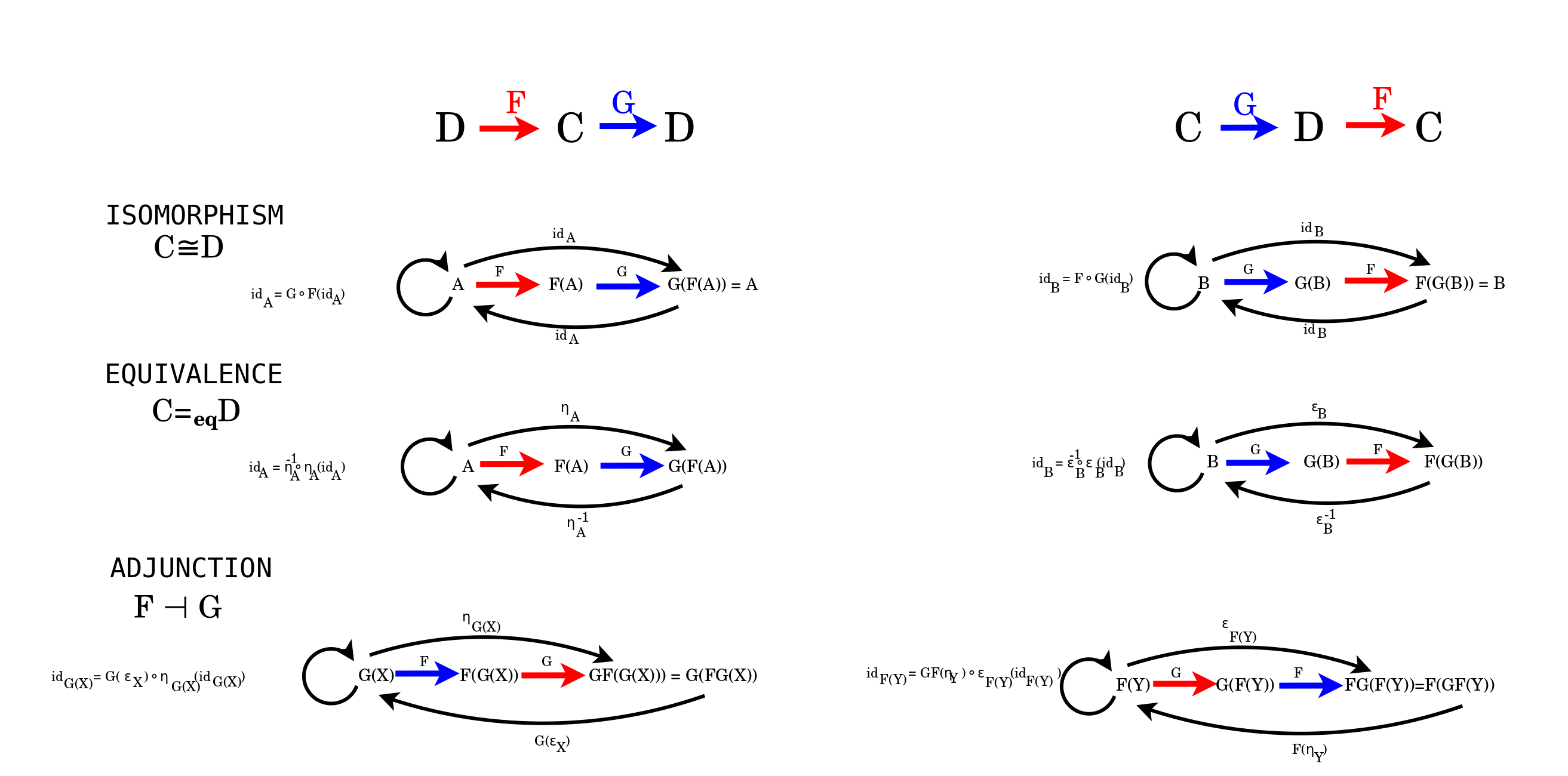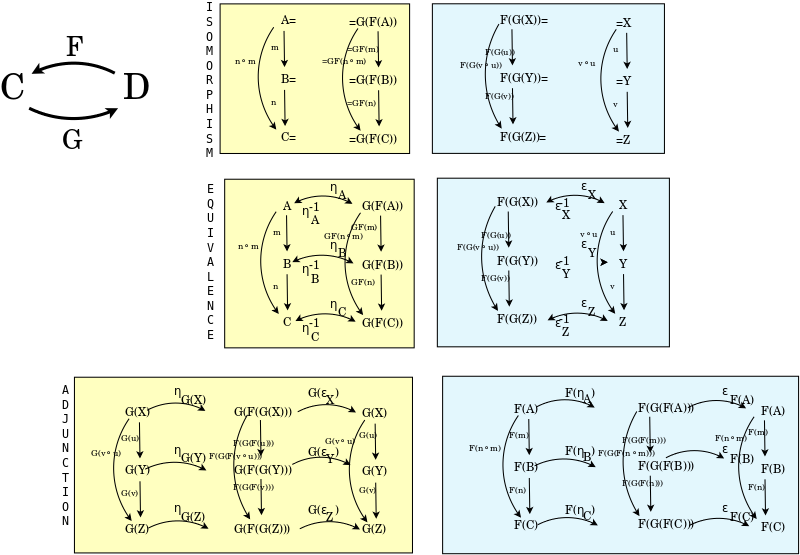Definitions of adjunction A functor {$F:D\to C$} is a left adjoint functor if for each object {$X$} in {$C$} there exists a universal morphism from {$F$} to {$X$}. For each object {$X$} in {$C$} there exists an object {$G(X)$} in {$D$} and a morphism {$ϵ_X: F(G(X))→X$} such that for every object {$Y$} in {$D$} and every morphism {$f: F(Y)→X$} there exists a unique morphism {$g:Y→G(X)$} with {$ϵ_X ∘ F(g) = f$}. A functor {$G:C → D$} is a right adjoint functor if for each object {$Y$} in {$D$}, there exists a universal morphism from {$Y$} to {$G$}. Spelled out, this means that for each object {$Y$} in {$D$}, there exists an object {$F(Y)$} in {$C$} and a morphism {$η_Y:Y → G(F(Y))$} such that for every object {$X$} in {$C$} and every morphism {$g:Y→G(X)$} there exists a unique morphism {$f:F(Y)→X$} with {$G(f)∘η_Y = g$}. Definitions Think of definitions of adjunctions as ratios of differences. Compare this with {$SU(2)$} and with cross-ratios. Check: the universal mapping property relates triangle identity to the identity. Adjunction's left and right parentheses are like particle and anti-particle, which can be created out of an identity morphism by way of the triangle identity. Adjunction's parenthesis in one world mirrors an identity in the other world. Adjunction's parentheses (unit and counit) and their respective worlds express reading and writing. Adjunction's parenthesis (unit or counit) is the boundary of a world (into or out of the image of the functor). There are four kinds of definitions for adjunction. This describes the formula for a perspective: Homset - balanced UMP for counit - step in ? UMP for unit - step out ? Triangle identities - consciousness Homset HomSet: The parenthesis establishes three zones: inside, border, outside, and likewise: outside, border, inside, and the transpose operation. HomSet {$\frac{A-B}{A'-B'}$} Universal mapping property Universal mapping property: Can always create a left parenthesis in the first morphism, as close to the object as you like. The Universal mapping property shows the asymmetry of F and G which is based on the asymmetry inherent in composition, which is unidirectional, rooted in an object. F and eta is covariant with composition, and G and epsilon is contravariant with composition. Universal mapping property {$\frac{A-B}{A-F(A)}$} An object in a comma category consists of an analogue and a correction. An object {$Y$} in {$\mathbf{C}$} has an analogue {$G(Y)$} in {$\mathbf{D}$} and an approximation {$F\circ G(Y)$} in {$\mathbf{C}$}. The correction {$\epsilon_Y:F\circ G(Y)\rightarrow Y$} corrects the approximation to yield the object. The correction {$\epsilon :F\circ G\rightarrow I_C$} is a natural transformation from the approximation functor to the identity functor. Triangle identity Triangle identity: Can cancel left and right parentheses if there is nothing between them. And can create a pair in empty space. The triangle identity just prepends eta_A and ignores postpending. Which is to say, we can postpend any G(B). Then it serves to remove the G from the B, and thus reverse the functor. Unit-counit triangle inequality {$\frac{A-F(A)}{G(F(A))-F(A)}$} and natural transformation {$\frac{A-B}{A-F(A)}$}. Unit-counit equations relate postcomposition and precomposition as with pushdown automata and the Yoneda lemma. Unit-counit adjunction defines the bar operation so as to define a triangle based on all three relevant dimensions.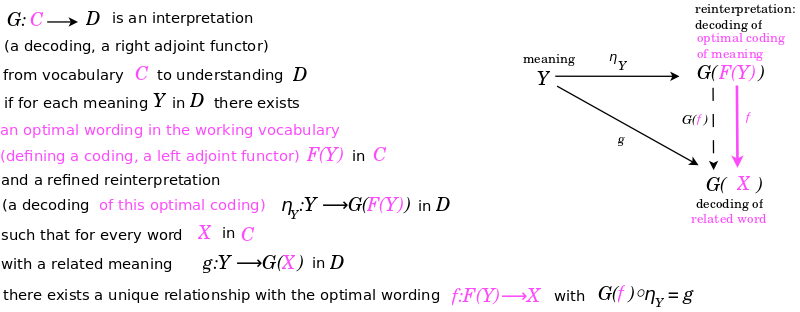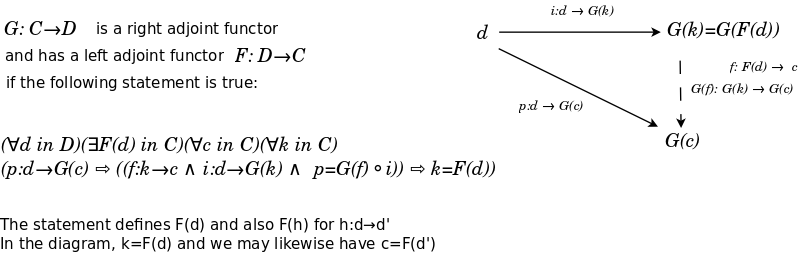Ideas about adjunctions Intuition about adjunction An adjoint functor is an approximate inverse, either as a pre-adjustment ("on the left") or as a post-adjustment ("on the right"). A functor is a perspective. An adjoint functor is an inverted perspective. An adjunction is defined by two different natural transformations that are separated by a functor (before and after) and a related functor (after and before). Adjunction relates a question and an answer. Adjunction is like extension of the domain, but in terms of structure: extension of structure. Polymorphism. Adjunction: G(F(x)) is the recalibration of x An adjoint functor, notably a trivial one, is a wholeness transformation, as described by Christopher Alexander. Adjunction can describe the details of restructuring of one structure by another structure and the abyss between them. Adjunction example: Lending a dollar bill and not getting back the same dollar bill, but an equivalent dollar bill. Adjunctions present the same information in different context. Information is given in the form of morphisms, not isolated objects. Morphisms are structure preserving maps, or more generally, validations that there is a way to go from one object to another. An adjunction relates contexts (categories) that are connected by patches (images of functors). Which is to say, an adjunction synchronizes two categories along a patch that may be all or part of the category on either side. Adjoint functors harmonize the semantics of information (which stays the same) and the syntax of context (which changes). A morphism synchronizes the clockwork of one representation with the clockwork of another representation. This synchronization is information. An adjunction matches this synchronization in one world (for one group) with the synchronization in another world (for another group). The matching is a natural isomorphism. Adjoint functors relate human and divine perspectives in trivial ways. Adjoint functors may relate to chains of perspectives of human's view and God's view. The identity triangles for units and counits are in terms of FGF. This reminds me of my thoughts on bisecting a view. FGF also shows the ambiguity of F, that it can be thought of as acting on the outside, F(GF->I), or on the inside (FG->I)_F Adjunction is like a half-inverted tube. There is an inversion point which can be changed, which can be freely chosen as desired, but cannot be either end. Adjunction is a relationship that lifts above the object and says what matters are the morphisms. But it does that with a pair of counterbalancing functors. Adjoint functors relate viewing forward and viewing backward as occurs with shifts of perspective in the foursome, fivesome, sixsome, sevensome. Adjoint strings Different kinds of adjunctions are different kinds of equivalences (or wholeness preserving transformations) such as freely generating or forgetting structure, inclusion and restriction - extending or restricting the domain, making copies or eliminating them. The identity functor can be thought of as the trivial functor for adjunctions involving dualities, two compatible functors. Free and forgetful "free" and "forgetful" are two opposite manifestations of "unrestricted". Like decreasing and increasing slack. Forgetfulness, freeness are properties with regard to the two ends of a morphism that restricts it or not, makes it less frequent or not. Tensor-Homset HomSet expresses choice. The left adjoint tensor product is like geometry ("homogeneity of choice") and the right adjoint homset is like algebra ("step by step"). The tensor-curry adjunction relates all and one, as between everything and anything, and between nothing and something. It allows for step-by-step algebra, as by the Yoneda lemma. {$f_*\dashv f^{-1}$} expresses perfect duality. The trivial functor is {$f_*$} which is aggregation. Adjoint string: Limit - diagonal {$\Delta$} - Colimit. This expresses my idea about external relations vs. internal structure. Adjoint strings express the operation +2, the alternation of Human perspective of God's perspective, and also thus the gap that starts with the foursome, grows with the fivesome, sixsome, sevensome, wear from that womb keep inserting new perspectives, flipping the direction, what it means to go beyond oneself. Classification of adjoint strings expresses the possiblities for harmonization of the semantics of information (which stays the same) and the syntax of context (which changes). Thus adjoint strings express the ways that a division into information and context can be understood within the context of divisions of everything, namely, within the context of the eightsome. Consider the adjoint string with seven functors, and the infinite adjoint string with period six. Divisions The twosome is modeled by the adjunction of the tensor product functor (opposites coexist - in parallel - commutative, internal duality A=A) and the homset functor (all is the same - in series - noncommutative, external duality A->B). Triangulated categories. The theory of derived functors can alternatively be expressed through that of derived categories, a special case of triangulated categories (or more precisely triangulated categories with chosen t-structures). Generalizing abelian categories, triangulated categories are merely additive categories but come equipped with a generalized notion of “exact sequences”, namely exact triangles. Much of classical homological algebra can be carried out on triangulated categories as well. [Wei94, § 10.1–§ 10.2] 21. Derived functors/categories (e.g., Tor, Ext, triangulated categories) The topics no. 17, “Homological algebra”, and no. 21, “Derived functors/categories (e.g., Tor, Ext, triangulated categories)”, are for all intents and purposes identical. Having multiple talks on it seems very much possible. Up to four are outlined below. Chain complexes. Chain complexes, usually of modules over a ring, are the prominent entities treated in homological algebra. Chain complexes form a category with chain maps as morphisms. Actually, they form a 2-category in the sense of higher order category theory with chain homotopies as second-order morphisms. The functor given by the operation of taking the homology of given chain complex is what gives the subject of homological algebra its name. It permits measuring to what degree a given chain complex fails to be exact (a property inspired by that of the same name for short or long sequences). [Wei94, § 1.1–§ 1.5], [Bla11, § 11.1] Left and right derived functors. A main interest of homological algebra is to study functors between categories of modules (or more generally abelian categories) via the homology of their compositions with other functors. One of the crucial tools for doing so is the concept of derived functors. The categories in question must have projective or injective objects, and enough of them at that, for this idea to work. The functor to be studied is then applied to projective or injective resolutions, chain complexes consisting of projective/injective objects, after which homology is taken. [Wei94, § 2.1–§ 2.5], [Bla11, § 11.2–§ 11.3] Consequences In the house of knowledge, the threesome creates a division of everything between local (algebra) and global (analysis) as an adjoint string. Facts about adjunctions Uniqueness Two right adjoints of a functor are naturally isomorphic. Focus on what the functor does to the morphisms. For example, a set function becomes a group homomorphism, and a group homomorphism becomes a set function. An inequality amongst the reals becomes an inequality amongst the integers, and vice versa. Relating adjunctions in category theory and linear algebra In linear/Lie algebra - Hermitian adjoint (Hermitian conjugate) - H_2=H_1 A**=A (A*)-1=(A-1)* Self-adjoint operator. {$K^{-1}K=I$}. Partitions (tableaux) are structures that relate to decomposition (factoring) of an item. Thus they relate to factorization of monoids. Thus it may be possible to show using adjunctions that they can't yield a graded involution, one that factors through. My thesis about eigenvalues of a matrix may involve an adjunction that relates a substitution right adjoint functor (for the matrix entries) and a left adjoint functor for reworking of nodes into edges. Correlation and causality Adjunction can relate correlation and causality. For they are two functors that are synchronized but they have different contexts. A correlation is like a forgetful functor in that it gives partial information. And causality is like a free construction in that it generates more information. Emergence Mathematically, emergence is described by free construction, thus left adjoints. Self-adjoint Hermitian adjoint The adjoint of an operator plays the role of the complex conjugate of a complex number. Self-adjoint operators play the role of the real numbers (being equal to their own "complex conjugate") and form a real vector space. They serve as the model of real-valued observables in quantum mechanics. The relation between self-adjoint (reals) and adjoint (complexes) gets repeated with the complexes and the quaternions, and with the quaternions and the octonions. If we think of the scalar product as summing over all elements of the group and thus ensuring group invariance, and if that scalar product is semilinear in the second argument, then inverting all of the group elements is the same as transposing and taking the complex conjugate of the matrix. Thus adjunction expresses this duality. If a representation is defined with regard to an orthonormal basis, then it is a unitary matrix. We can think of a unitary matrix as expressing that a representation is defined with regard to an orthonormal basis defined with regard to the scalar product. Self-adjoint means that it is "observable" because observability requires being able to approach it from both directions of causality, forwards and backwards, as per the critical point - the ambiguous point - of the fivesome. Organization of knowledge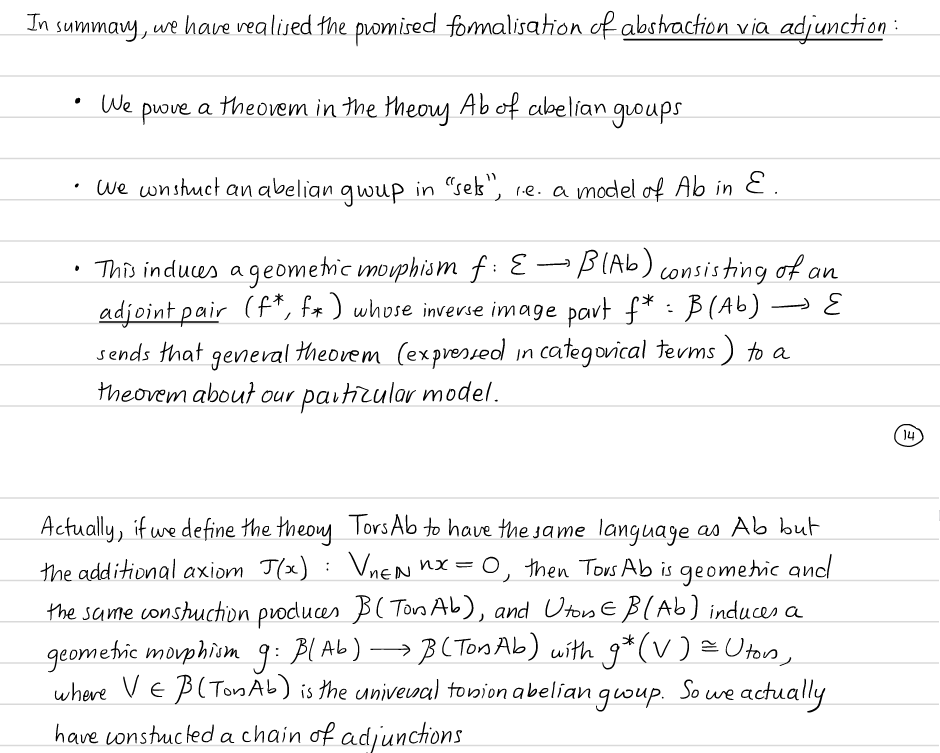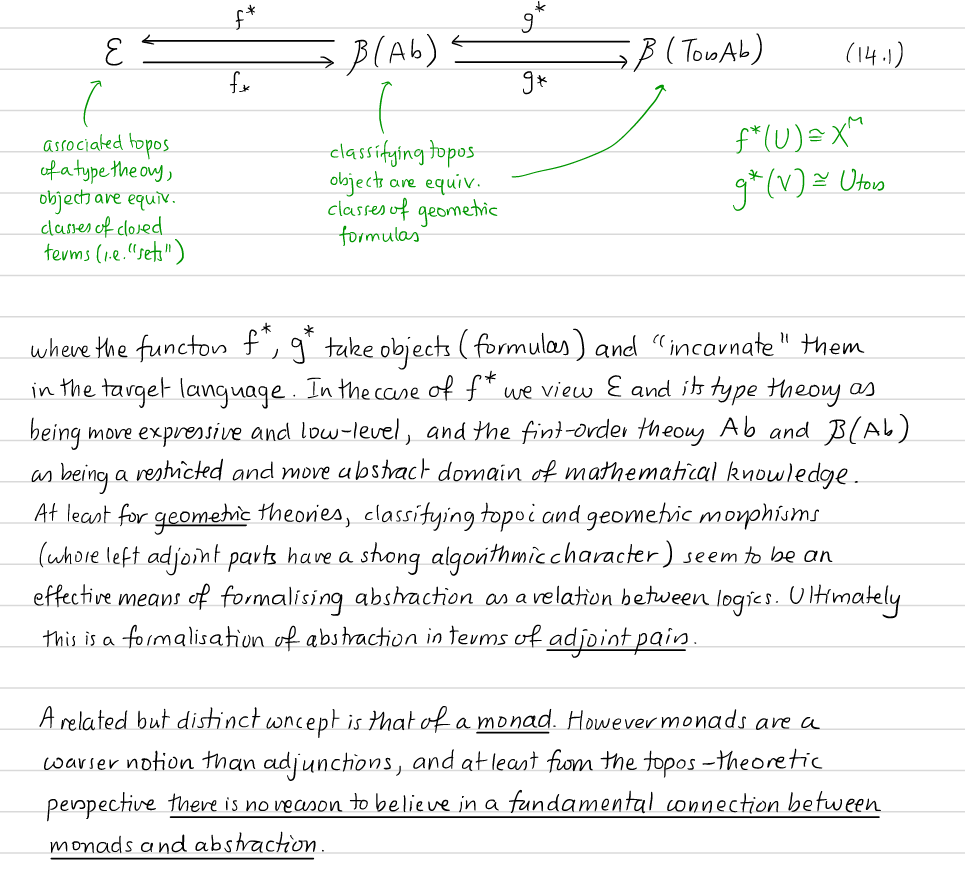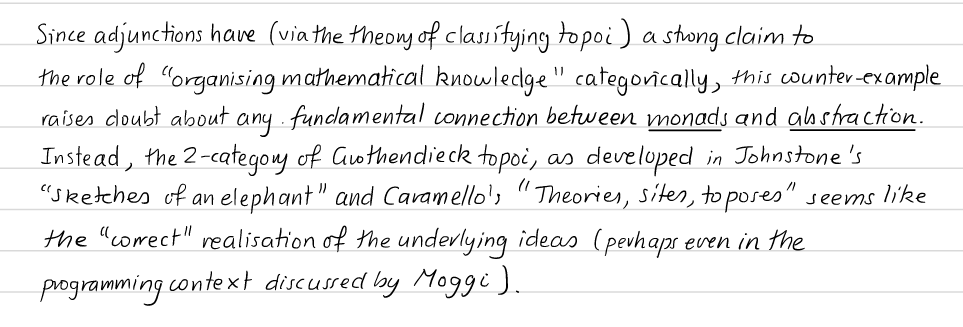Šis puslapis paskutinį kartą keistas November 28, 2021, at 06:45 PM